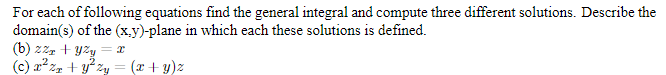# For each of following equations find the general integral and compute three different solutions. Describe thedomain(s) of the (x.y)-plane in which each these solutions is defined.(b) zzz + yzy(c) a² zr + y² zy = (x + y)z

Question
1 viewshelp_outlineImage TranscriptioncloseFor each of following equations find the general integral and compute three different solutions. Describe the domain(s) of the (x.y)-plane in which each these solutions is defined. (b) zzz + yzy (c) a² zr + y² zy = (x + y)z fullscreen
check_circle

Step 1

We’ll answer the first question since the exact one wasn’t

specified. Please submit a new question specifying the one you’d like answered.

Step 2

b)Given that the partial differential equation

Step 3

Comparing this equation with the general the ...

### Want to see the full answer?

See Solution

#### Want to see this answer and more?

Solutions are written by subject experts who are available 24/7. Questions are typically answered within 1 hour.*

See Solution
*Response times may vary by subject and question.
Tagged in